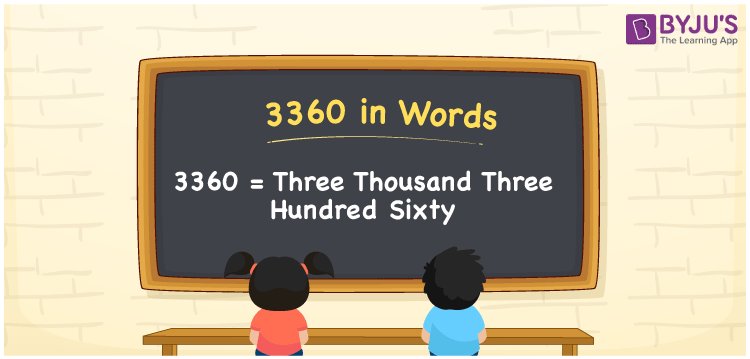# 3360 in Words

We can write 3360 in words as Three thousand three hundred sixty. Conversion of the number 3360 into words can be done quickly with the help of a place value chart. However, the word form of 3360 is nothing but the number name. For example, the number name for 3 is Three. Similarly, the number name for 3360 is Three thousand three hundred sixty. Let’s understand how to derive the word form for 3360 in detail here in this article.

 3360 in words Three thousand three hundred sixty Three thousand three hundred sixty in Numbers 3360

## 3360 in English Words

We generally write numbers in words using the English alphabet. So, we can read 3360 in English as “Three thousand three hundred sixty”.## How to Write 3360 in Words?

The below table shows the place values for all the four digits of the number 3360. This can be used to derive the word form of the given number, i.e. 3360.

 Thousands Hundreds Tens Ones 3 3 6 0

Here, ones = 0, tens = 6, hundreds = 3, thousands = 3

These values can be written as:

3 × Thousand + 3 × Hundred + 6 × Ten + 0 × One

= 3 × 1000 + 3 × 100 + 6 × 10 + 0 × 1

= 3000 + 300 + 60

= Three thousand + Three hundred + Sixty

= Three thousand three hundred sixty

Therefore, 3360 in words = Three thousand three hundred sixty.

As we know, 3360 is a natural number that precedes 3361 and succeeds 3359.

3360 in words – Three thousand three hundred sixty

Is 3360 an odd number? – No

Is 3360 an even number? – Yes

Is 3360 a perfect square number? – No

Is 3360 a perfect cube number? – No

Is 3360 a prime number? – No

Is 3360 a composite number? – Yes

## Frequently Asked Questions on 3360 in Words

Q1

### How do you write 3360 in English words?

In English words, we can write the number 3360 as Three thousand three hundred sixty.
Q2

### How to write Rs. 3360 in words on a cheque?

On a cheque, we generally write Rs. 3360 in words as “Three thousand three hundred sixty rupees only”.
Q3

### Express the answer for 1 + 3360 in words.

1 + 3360 = 3361 Therefore, 1 + 3360 is equal to Three thousand three hundred sixty-one.